# Rotten Oranges

Problem Statement: Given a grid of dimension N x M where each cell in the grid can have values 0, 1, or 2 which has the following meaning:

0: Empty cell

1: Cells have fresh oranges

2: Cells have rotten oranges

We have to determine what is the minimum time required to rot all oranges. A rotten orange at index [i,j] can rot other fresh oranges at indexes [i-1,j], [i+1,j], [i,j-1], [i,j+1] (up, down, left and right) in unit time.

Pre-req: Graph traversal techniques, Queue STL

Examples:

Example 1:

Input:

Output: 4

Explanation: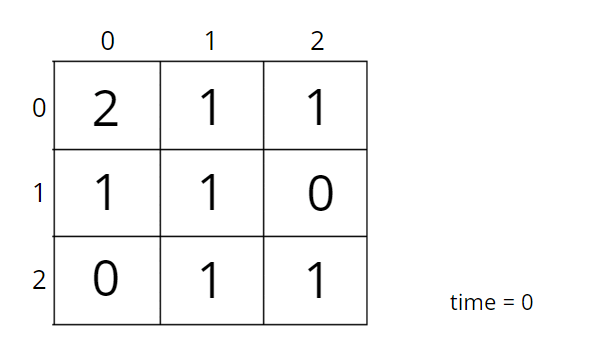Example 2:

Output: 1

Explanation: There are 3 rotten oranges in this example. Rotting of oranges happens simultaneously.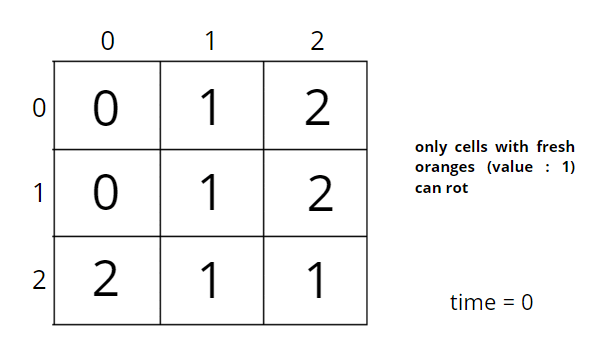To understand a better step-by-step process of simultaneous rotting consider the following illustration: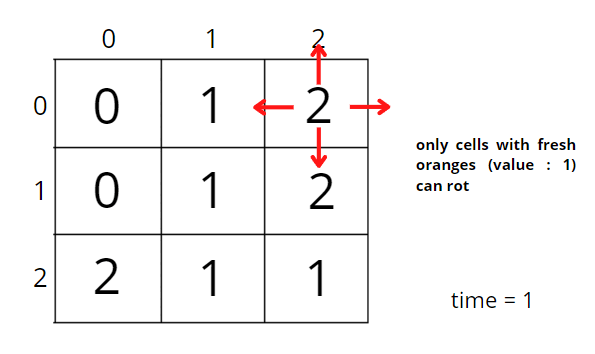### Solution

Disclaimer: Don’t jump directly to the solution, try it out yourself first.

### Intuition:

A rotten orange at index [i,j] can rot other fresh oranges at indexes [i-1,j], [i+1,j], [i,j-1], [i,j+1] (up, down, left and right) in unit time, i.e., in 4 directions.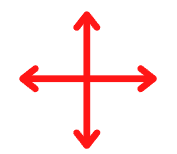The question arises of which algorithm to use.

A rotten orange can rot fresh orange neighbours that are at a distance of 1 or at the same level. It means each of them got rotten at a similar level or stage, implying we need to visit the same level at the same time. Hence, level-wise traversal is BFS traversal.

If we use DFS traversal then all neighbouring fresh oranges will be visited depth-wise. But here it is not the case to rot all the oranges, we need to find the minimum time to rot them all, which is possible only when we are in neighbouring directions at an equal pace. We want to rotten them simultaneously.

So, BFS traversal will be used to solve this problem.

Approach:

Initial configuration:

• Queue: contains a couple of starting points that will depend on the number of rotten oranges present initially.
• Visited array: is of the same size as the grid. The visited cell represents rotten orange.

The algorithm steps are as follows:

• For BFS traversal, we need a queue data structure and a visited array. Create a replica of the given array, i.e., create another array of the same size and call it a visited array. We can use the same matrix, but we will avoid alteration of the original data.
• The pairs of cell number and initial time, i.e., <<row, column>, time> will be pushed in the queue and marked as visited (represents rotten) in the visited array. For example, ((2,0), 0) represents cell (2, 0) and initial time 0.
• While BFS traversal, pop out an element from the queue and travel to all its neighbours. In a graph, we store the list of neighbours in an adjacency list but here we know the neighbours are in 4 directions.
• We go in all 4 directions and check for valid unvisited fresh orange neighbours. To travel 4 directions we will use nested loops, you can find the implementation details in the code.
• BFS function call will make sure that it starts the BFS call from each rotten orange cell, and rotten all the valid fresh orange neighbours and puts them in the queue with an increase in time by 1 unit. Make sure to mark it as rotten in the visited array.
• Pop-out another rotten orange from the queue and repeat the same steps until the queue becomes empty.
• Add a counter variable to store the maximum time and return it. If any of the fresh was not rotten in the visited array then return -1.

Consider the following example to understand how BFS traverses the cells and rotten the oranges accordingly.

`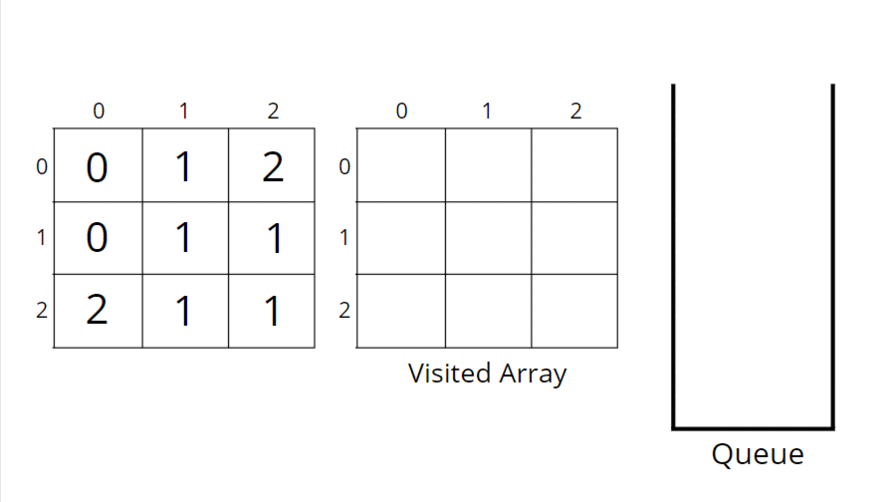`

How do set boundaries for 4 directions?

The 4 neighbours will have the following indexes: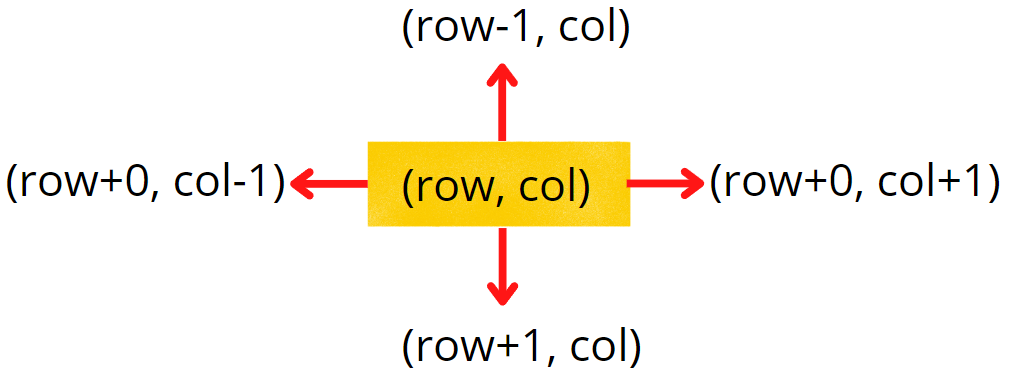Now, either we can apply 4 conditions or follow the following method.

From the above image, it is clear that the delta change in a row is -1, +0, +1, +0. Similarly, the delta change in column is 0, +1, +0, -1.  So we can apply the same logic to find the neighbours of a particular pixel (<row, column>).

Code:

## C++ Code

``````#include<bits/stdc++.h>

using namespace std;

class Solution {
public:
//Function to find minimum time required to rot all oranges.
int orangesRotting(vector < vector < int >> & grid) {
// figure out the grid size
int n = grid.size();
int m = grid.size();

// store {{row, column}, time}
queue < pair < pair < int, int > , int >> q;
int vis[n][m];
int cntFresh = 0;
for (int i = 0; i < n; i++) {
for (int j = 0; j < m; j++) {
// if cell contains rotten orange
if (grid[i][j] == 2) {
q.push({{i, j}, 0});
// mark as visited (rotten) in visited array
vis[i][j] = 2;
}
// if not rotten
else {
vis[i][j] = 0;
}
// count fresh oranges
if (grid[i][j] == 1) cntFresh++;
}
}

int tm = 0;
// delta row and delta column
int drow[] = {-1, 0, +1, 0};
int dcol[] = {0, 1, 0, -1};
int cnt = 0;

// bfs traversal (until the queue becomes empty)
while (!q.empty()) {
int r = q.front().first.first;
int c = q.front().first.second;
int t = q.front().second;
tm = max(tm, t);
q.pop();
// exactly 4 neighbours
for (int i = 0; i < 4; i++) {
// neighbouring row and column
int nrow = r + drow[i];
int ncol = c + dcol[i];
// check for valid cell and
// then for unvisited fresh orange
if (nrow >= 0 && nrow < n && ncol >= 0 && ncol < m &&
vis[nrow][ncol] == 0 && grid[nrow][ncol] == 1) {
// push in queue with timer increased
q.push({{nrow, ncol}, t + 1});
// mark as rotten
vis[nrow][ncol] = 2;
cnt++;
}
}
}

// if all oranges are not rotten
if (cnt != cntFresh) return -1;

return tm;

}
};

int main() {

vector<vector<int>>grid{{0,1,2},{0,1,2},{2,1,1}};
Solution obj;
int ans = obj.orangesRotting(grid);
cout << ans << "\n";

return 0;
}``````

Output: 1

Time Complexity: O(NxM + NxMx4) ~ O(N x M), For the worst case, all of the cells will have fresh oranges, so the BFS function will be called for (N x M) nodes and for every node, we are traversing for 4 neighbours, it will take O(N x M x 4) time.

Space Complexity ~ O(N x M), O(N x M) for copied input array and recursive stack space takes up N x M locations at max.

## Java Code

``````import java.util.*;
class Solution {
//Function to find minimum time required to rot all oranges.
public int orangesRotting(int[][] grid) {
// figure out the grid size
int n = grid.length;
int m = grid.length;
// n x m
Queue < Pair > q = new LinkedList < > ();
// n x m
int[][] vis = new int[n][m];
int cntFresh = 0;

for (int i = 0; i < n; i++) {
for (int j = 0; j < m; j++) {
// if cell contains rotten orange
if (grid[i][j] == 2) {
q.add(new Pair(i, j, 0));
// mark as visited (rotten) in visited array
vis[i][j] = 2;
}
// if not rotten
else {
vis[i][j] = 0;
}

// count fresh oranges
if (grid[i][j] == 1) cntFresh++;
}
}

int tm = 0;
// delta row and delta column
int drow[] = {-1, 0, +1, 0};
int dcol[] = {0, 1, 0, -1};
int cnt = 0;

// until the queue becomes empty
while (!q.isEmpty()) {
int r = q.peek().row;
int c = q.peek().col;
int t = q.peek().tm;
tm = Math.max(tm, t);
q.remove();
// exactly 4 neighbours
for (int i = 0; i < 4; i++) {
int nrow = r + drow[i];
int ncol = c + dcol[i];
// check for valid coordinates and
// then for unvisited fresh orange
if (nrow >= 0 && nrow < n && ncol >= 0 && ncol < m &&
vis[nrow][ncol] == 0 && grid[nrow][ncol] == 1) {
// push in queue with timer increased
q.add(new Pair(nrow, ncol, t + 1));
// mark as rotten
vis[nrow][ncol] = 2;
cnt++;
}
}
}

// if all oranges are not rotten
if (cnt != cntFresh) return -1;
return tm;
}

public static void main(String[] args) {
int[][] grid =  {{0,1,2},{0,1,2},{2,1,1}};

Solution obj = new Solution();
int ans = obj.orangesRotting(grid);
System.out.println(ans);
}

}

class Pair {
int row;
int col;
int tm;
Pair(int _row, int _col, int _tm) {
this.row = _row;
this.col = _col;
this.tm = _tm;
}
}``````

Output: 1

Time Complexity: O(NxM + NxMx4) ~ O(N x M), For the worst case, all of the cells will have fresh oranges, so the BFS function will be called for (N x M) nodes and for every node, we are traversing for 4 neighbours, it will take O(N x M x 4) time.

Space Complexity ~ O(N x M), O(N x M) for copied input array and recursive stack space takes up N x M locations at max.

Special thanks to Vanshika Singh Gour for contributing to this article on takeUforward. If you also wish to share your knowledge with the takeUforward fam, please check out this articleIf you want to suggest any improvement/correction in this article please mail us at [email protected]